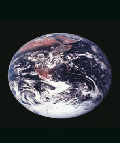What's NewEinstein's Theory. How do we know it is true. Just because energy = mass * velocity of light, the equation says that  the mass at the speed of light is infinitely large. No one has ever travelled at the speed of light so how can we prove it. Can we trust the theory without
 proving it?   The theory says that the mass of an object will be infinitely large, the length of the object will be infinitely small and time is infinitely large. Einstein then says that to travel faster than the speed of light is impossible.   If we address each question in turn on mass,
 length and time, is it impossible?